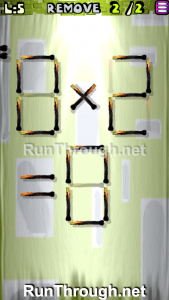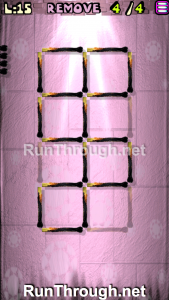# Move the Matches Puzzles Episode 5 Level 1-20 Walkthrough

Walkthrough for all the solutions and answers to the game Move the Matches Puzzles by K-Lab. Get the app on the Google Play Store to play along if you do not already have it. This is for episode 5, levels 1 through 20.

The format for the walkthrough images are as follows:  we will be showing the final image of the Matches Puzzle. This will allow you to see where everything goes, and to know for sure that the puzzle works.

###Instructions: Remove 5 matches to get 7 squares, all equal

###Instructions: Move 1 match to correct the equation

Solution: 3×5=15

###Instructions: Remove 2 matches to correct the equation

Solution: 5+5=10

###Instructions: Remove 6 matches to get 4 squares

###Instructions: Remove 2 matches to correct the equation

Solution: 0x2=0

###Instructions: Move 2 matches to get 3 squares

###Instructions: Move 1 match to correct the equation

Solution: 6×9=54

###Instructions: Move 3 matches to get 5 squares

###Instructions: Add 2 matches to correct the equation

Solution: 6×8=48

###Instructions: Move 4 matches to get 4 triangles

###Instructions: Add 2 matches to correct the equation

Solution: 9-2=7

###Instructions: Remove 2 matches to correct the equation

Solution: 6×6=36

###Instructions: Move 4 matches to get 2 squares

###Instructions: Move 2 matches to correct the equation

Solution: 8×8=64

###Instructions: Remove 4 matches to get 5 squares

###Instructions: Remove 2 matches to correct the equation

Solution: 6-5=1

###Instructions: Move 1 match to correct the equation

Solution: 4×8=32

###Instructions: Remove 2 matches to correct the equation

Solution: 6-1=5

###Instructions: Add 2 matches to correct the equation

Solution: 8+4=12

### Level 20Instructions: Remove 3 matches to get 4 squares